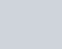Solve this following
Question:

Prove that

$\int_{0}^{1} x(1-x)^{5} d x=\frac{1}{42}$

Solution: